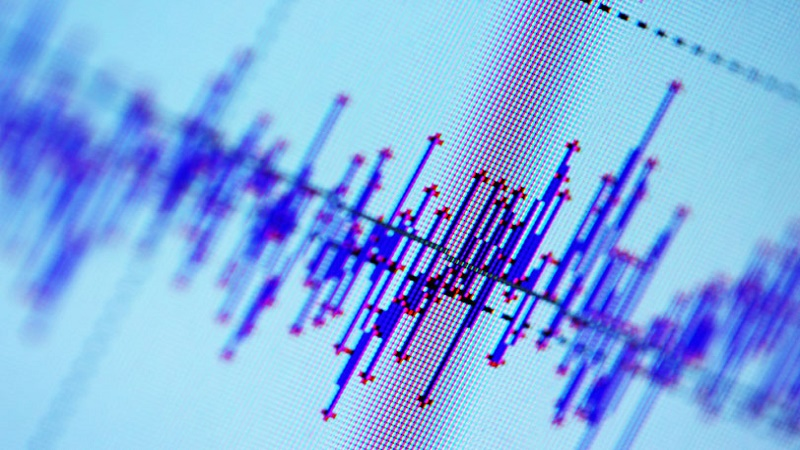Signals and Systems - EC8352, EC6303

Online Study Material, Lecturing Notes, Assignment, Reference, Wiki and important questions and answersCLASSIFICATION OF SIGNALS AND SYSTEMS

=> Classification of Signals and Systems: Introduction
=> Continuous-time and discrete-time systems
=> Elementary Signals
=> Classification of CT and DT signals
=> Classification of system
=> Sample Solved Problems: Classification of Signals and Systems
=> Important Short Questions and Answers: Classification of Signals and Systems

ANALYSIS OF CONTINUOUS TIME SIGNALS

=> Fourier series analysis
=> Continuous Time Fourier Transform
=> Inverse Transforms
=> Parseval’s theorem
=> Laplace Transform
=> Properties of ROC of Laplace Transform
=> Important Short Questions and Answers: Analysis of Continuous Time Signals

LINEAR TIME INVARIANT CONTINUOUS TIME SYSTEMS

=> Linear Time Invariant -Continuous Time Systems
=> Important Short Questions and Answers: Linear Time Invariant, Continuous Time Systems

ANALYSIS OF DISCRETE TIME SIGNALS

=> Analysis of Discrete Time Signals
=> Important Short Questions and Answers: Analysis of Discrete Time Signals

LINEAR TIME INVARIANT

=> Linear Time Invariant Discrete Time Systems
=> Sample Problems and Important Short Answers: Linear Time Invariant Discrete Time Systems

EC6303 Signals and Systems - Anna University 2013 Regulation Syllabus - Download Pdf
EC8352 Signals and Systems - Anna University 2017 Regulation Syllabus - Download Pdf